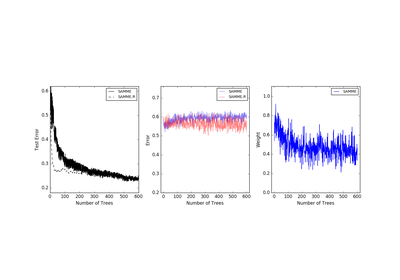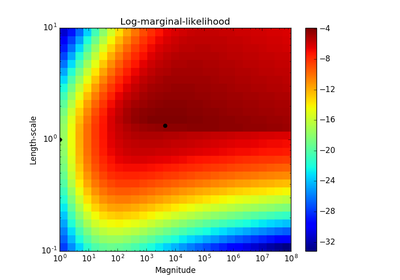# `sklearn.metrics`.accuracy_score¶

`sklearn.metrics.``accuracy_score`(y_true, y_pred, normalize=True, sample_weight=None)[source]

Accuracy classification score.

In multilabel classification, this function computes subset accuracy: the set of labels predicted for a sample must exactly match the corresponding set of labels in y_true.

Read more in the User Guide.

Parameters: y_true : 1d array-like, or label indicator array / sparse matrix Ground truth (correct) labels. y_pred : 1d array-like, or label indicator array / sparse matrix Predicted labels, as returned by a classifier. normalize : bool, optional (default=True) If `False`, return the number of correctly classified samples. Otherwise, return the fraction of correctly classified samples. sample_weight : array-like of shape = [n_samples], optional Sample weights. score : float If `normalize == True`, return the correctly classified samples (float), else it returns the number of correctly classified samples (int). The best performance is 1 with `normalize == True` and the number of samples with `normalize == False`.

Notes

In binary and multiclass classification, this function is equal to the `jaccard_similarity_score` function.

Examples

```>>> import numpy as np
>>> from sklearn.metrics import accuracy_score
>>> y_pred = [0, 2, 1, 3]
>>> y_true = [0, 1, 2, 3]
>>> accuracy_score(y_true, y_pred)
0.5
>>> accuracy_score(y_true, y_pred, normalize=False)
2
```

In the multilabel case with binary label indicators: >>> accuracy_score(np.array([[0, 1], [1, 1]]), np.ones((2, 2))) 0.5

## Examples using `sklearn.metrics.accuracy_score`¶